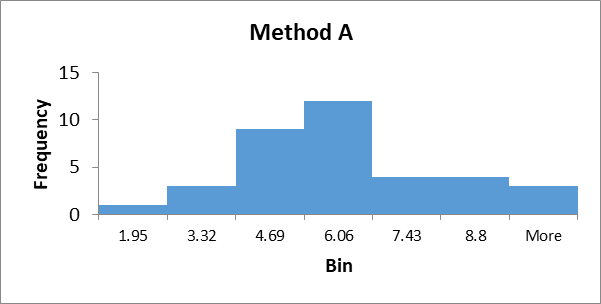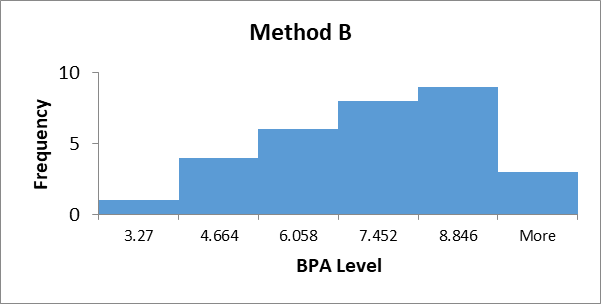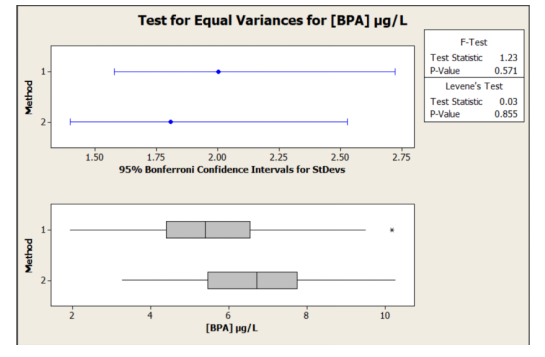# BIO2003 Hypothetical Test With Respect To Levels Of Bisphenol A Homework AnswerPages: 4Words: 890

## Question :

Course ID: BIO2003/BIO2005/BMS2007/BMS2075/BMS2475
Introduction

The UK’s Committee on Toxicity of Chemicals in Food launched a research that investigates levels of BPA in two different fruit juice carton packaging techniques (A and B), both using cardboard coated with processes involving BPA. In order to test whether the fruit juice quality was adversely affected by the packaging process, an experiment was undertaken comparing the level of BPA from the same batch of fruit juice which was randomly allocated to cartons made using the two techniques. A total of 67 cartons of fruit juice were prepared, 36 using method A and 31 using method B.  After being kept in identical conditions (same time, same size/shaped carton, same volume of fruit juice), the cartons were opened and the fruit juice analysed for BPA using High Performance Liquid Chromatography (HPLC) via the agency of an accredited analytical chemistry laboratory.

The experimental hypothesis was that BPA was leaching out of the packaging material and entering the fruit juice and that there would be a difference between the two packaging processes with respect to the BPA level.

Problem

The following report aims at conducting hypothetical test with respect to levels of Bisphenol A (BPA) [IUPAC name 4,4'-(propane-2,2-diyl)diphenol] which is a chemical that is widely used in the manufacture of inert coatings of many types of food packaging. In concentrated form, there is evidence that BPA has hormone-like properties e.g. mimicking oestrogen. This has raised concerns about its presence in consumer products.

The Committee needs to know what is the evidence, based on the level of BPA in the fruit juice, whether there is a significant difference between the two carton packaging processes.

Dataset

The data comprises of 67 analysed packages of fruit juice. Three variables are presented below: the sample identifier number, the BPA concentration (in µg/L), and the type of packaging process/method. The data table is available as appendix.

Statistical Testing

The above mentioned scenario comprises of two types of methods of packaging: A and B. The provided data comprises of BPA levels in each fruit package under either type of methods. Hence, in order to compare the level of BPA in Method A as compared to Method B, we need to use a statistical test to compare means.

The selected test is T-test for Two Independent Means. This is because the test involves two datasets or samples: method A and Method B. Further, the data observations for each of the method is independent of each other. Hence, the test can be used.

Assumptions of the Selected Test

Before the T-test for Two Independent Means can be used, the dataset needs to be checked if it meets the assumptions for the test. Following are the assumptions for a T-test for Two Independent Means:

1. Normal Distribution: The selected test assumes that the dependent variable is approximately normally distributed within each group. Hence, in the given scenario, we need to test if the BPA levels in juice packages are normally distributed (approximately) for each of the Method A and Method B. This can be done by creating histogram for the data under each of the method. The output is as follows:It can be seen that both the sample datasets are approximately normally distributed. The group sizes are also similar at 36 observations under Method A and 31 observations under Method B.

2. Homogeneity of Variance: The selected test also assumes that the variances are homogeneous in nature. For testing the homogeneity of variance, F-Test for two sample variances was conducted as follows:It can be seen that the p-value of 0.2854 is way higher than assumed significance level of 0.05. Hence, we can say that we do not have sufficient evidence to reject the null hypothesis. Hence, the variances of the two data sets are homogeneous.

Conducting the Selected Test

In the above section, we checked that the assumptions required for conducting a t-test for two independent means are being satisfied in the given scenario of 67 juice packages packed through either Method A or Method B. Now, we can conduct the t-test to compare the mean BPA level in juice package packed through Method A and the mean BPA level in juice package packed through Method B.

The hypothesis for the test is as follows:

• The Null Hypothesis is that the mean BPA level under Method A is same as mean BPA level under method B or H0: µA = µB
• The Alternative Hypothesis is that the mean BPA level under Method A is not same as mean BPA level under method B or H1: µA ≠ µB

The significance level is:

We need to assume a significance level such that the statistical test can be concluded. Hence, we assume an alpha of α = 0.05. This indicates that the certainty that the test conclusion is true is 95%.

The actual test was conducted using the inbuilt data tools in Microsoft Excel. The test output is as follows:

 t-Test: Two-Sample Assuming Equal Variances [BPA] µg/L Method A [BPA] µg/L Method B Mean 5.519 6.572 Variance 4.010 3.267 Observations 36 31 Pooled Variance 3.667 Hypothesized Mean Difference - Df 65.000 t Stat -2.245 P(T<=t) one-tail 0.014 t Critical one-tail 1.669 P(T<=t) two-tail 0.028 t Critical two-tail 1.997

From above, it can be seen that the calculated t-stat is 2.245. Also, the two-tailed p-value is 0.028.

Now, if the p-value is lower than the assumed significance level, then we have sufficient statistical evidence to reject the null hypothesis. However, if the p-value is higher than the assumed significance level, then we do not have sufficient evidence to reject the null hypothesis.

From the above output, it can be seen that the p-value of 0.028 is lower than the assumed significance level of 0.05. Hence, we have evidence to reject the null hypothesis.

The statistical test conclusion is:

Since the p-value was lower than the assumed significance level, we have sufficient evidence to reject the null hypothesis.

Hence, we can conclude that the mean level of BPA in juice packages packed under method A vary significantly from the mean level of BPA in juice packages packed under Method B. It also seems that the mean BPA level of BPA in juice packages packed under method A is lower than the mean level of BPA in juice packages packed under Method B.

Appendix

BPA concentrations (µg/L) in fruit juice following packaging by two methods

ID      [BPA] µg/L     Method

19.49A

25.50A

35.59A

43.47A

55.87A

66.05A

79.17A

81.95A

92.79A

108.21A

115.33A

124.43A

134.84A

143.94A

152.22A

164.41A

175.26A

185.55A

194.39A

204.47A

216.39A

224.45A

236.19A

244.09A

258.39A

265.89A

276.82A

285.44A

297.47A

301.98A

316.58A

324.15A

335.34A

347.63A

3510.17A

364.77A

373.27B

3810.24B

393.89B

408.49B

415.91B

426.71B

435.54B

447.88B

453.62B

467.75B

475.46B

486.94B

497.36B

506.41B

517.60B

524.73B

534.95B

546.33B

559.41B

563.79B

577.64B

587.72B

597.78B

606.30B

617.81B

627.50B

635.61B

643.95B

659.72B

667.14B

676.29B

Introduction

The UK’s Committee on Toxicity of Chemicals in Food launched a research that investigates levels of BPA with respect to two packaging techniques used for packing fruit juice cartons. The common factor for both the packaging techniques is that the cardboard coating comprises of BPA. The BPA is suspected to leach out from the material and enter the fruit juice contents and since BPA is known to have multiple harmful effects, the situation is serious.

To test whether there is difference in BPA levels in fruit juice packaged through different packaging techniques, a batch of 67 fruit juice cartons was distributed in a random manner to either packaging techniques out of Technique A and Technique B. All the packed cartons were kept in exactly same situations (time, size, shape, volume etc. being same). Then the cartons were opened, and sample of fruit juice was taken from each of the 67 cartons. The level of BPA was checked using High Performance Liquid Chromatography (HPLC) through the agency of an accredited analytical chemistry laboratory.

Problem

The following report aims at conducting hypothetical test with respect to levels of Bisphenol A (BPA) [IUPAC name 4,4'-(propane-2,2-diyl)diphenol] which is a chemical that is used extensively for  the production of inert coatings of several different food packaging. When highly concentrated, BPA is known to mimic hormone-like properties and hence, there are concerns if it is present in consumer products.

The report will be performing hypothetical testing to analyse if there is significant difference in level of BPA in fruit juice from cartons packed through packing technique A versus packing technique B.

Dataset

The data comprises of 67 analysed packages of fruit juice with three variables being the sample identifier number, the BPA concentration (in µg/L), and the type of packaging process/method. The data table is available as appendix.

Statistical Testing

The above mentioned scenario comprises of two types of methods of packaging: A and B. The provided data comprises of BPA levels in each fruit package under either type of methods. Hence, in order to compare the level of BPA in Method A as compared to Method B, we need to use a statistical test to compare means.

The selected test is T-test for Two Independent Means. This is because the test involves two datasets or samples: method A and Method B. Further, the data observations for each of the method is independent of each other. Hence, the test can be used.

Assumptions of the Selected Test

Before the T-test for Two Independent Means can be used, the dataset needs to be checked if it meets the assumptions for the test. Following are the assumptions for a T-test for Two Independent Means:

1. Normal Distribution: The selected test assumes that the dependent variable is approximately normally distributed within each group. Hence, in the given scenario, we need to test if the BPA levels in juice packages are normally distributed (approximately) for each of the Method A and Method B. This can be done by creating histogram for the data under each of the method. The output is as follows:It can be seen that both the sample datasets are approximately normally distributed. The group sizes are also similar at 36 observations under Method A and 31 observations under Method B.

1. Homogeneity of Variance: The selected test also assumes that the variances are homogeneous in nature. For testing the homogeneity of variance, test for equal variances was performed in Minitab as follows:It can be seen that the p-value of F-Test is 0.571 and p-value of Levene’s Test is 0.855 and both are way higher than assumed significance level of 0.05. Hence, we can say that we do not have sufficient evidence to reject the null hypothesis. Hence, the variances of the two data sets are homogeneous.

Conducting the Selected Test

In the above section, we checked that the assumptions required for conducting a t-test for two independent means are being satisfied in the given scenario of 67 juice packages packed through either Method A or Method B. Now, we can conduct the t-test to compare the mean BPA level in juice package packed through Method A and the mean BPA level in juice package packed through Method B.

The hypothesis for the test is as follows:

• The Null Hypothesis is that the mean BPA level under Method A is same as mean BPA level under method B or H0: µA = µB
• The Alternative Hypothesis is that the mean BPA level under Method A is not same as mean BPA level under method B or H1: µA ≠ µB

The significance level is:

We need to assume a significance level such that the statistical test can be concluded. Hence, we assume an alpha of α = 0.05. This indicates that the certainty that the test conclusion is true is 95%.

The actual test was conducted using the two-sample t-test in Minitab. The test output is as follows:From above, it can be seen that the calculated t-stat is -2.26. Also, the two-tailed p-value is 0.027.

Now, if the p-value is lower than the assumed significance level, then we have sufficient statistical evidence to reject the null hypothesis. However, if the p-value is higher than the assumed significance level, then we do not have sufficient evidence to reject the null hypothesis.

From the above output, it can be seen that the p-value of 0.027 is lower than the assumed significance level of 0.05. Hence, we have evidence to reject the null hypothesis.

The statistical test conclusion is:

As the p-value was lower than the anticipated significance level, we have adequate evidence to reject the null hypothesis.

Hence, it is clear that the mean level of BPA in juice packages packed under method A vary significantly from the mean level of BPA in juice packages packed under Method B. It also seems that the mean BPA level of BPA in juice packages packed under method A is lower than the mean level of BPA in juice packages packed under Method B.

Tags: